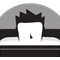# Using Geometry.fromJSON() gives unexpected results

299
2
05-07-2020 07:42 AMbyEsri Contributor

I am trying to use Geometry.fromJSON() in the ArcGIS JSAPI 4.x to return a Point or an Extent geometry and I am getting some unexpected results.

Here is what I have tried.

```const point = Geometry.fromJSON({x: 10, y: 10, spatialReference: {wkid: 4326}})
const point = Geometry.fromJSON({type: "point", x: 10, y: 10, spatialReference: {wkid: 4326}})
const point = Geometry.fromJSON(JSON.stringify({x: 10, y: 10, spatialReference: {wkid: 4326}}))
const point = Geometry.fromJSON({"x": 10, "y": 10, "spatialReference": {"wkid": 4326}})
const point = Geometry.fromJSON('{"x": 10, "y": 10, "spatialReference": {"wkid": 4326}}')
...
console.log(JSON.stringify(point.toJSON()))```

Here is the result in the console.

`{"spatialReference":{"wkid":4326}}`

Should I be able to get a Point geometry with the JSON I am using with Geometry.fromJSON()?

My goal would be to do the following...

`mapView.goTo(Geometry.fromJSON({....});`

...using valid JSON for a Point or an Extent.

2 RepliesbyEsri Contributor

Hi Eric, I would try using Point.fromJSON() instead.byEsri Contributor

Anne,

Thank you for the reply. I did notice that worked, and I forgot to add that detail to my post. The JSON will be describing a point or an extent so I was hoping that Geomery.fromJSON() would be able to create a Point from point JSON and an Extent from extent JSON. Am I using Geometry.fromJSON() incorrectly?